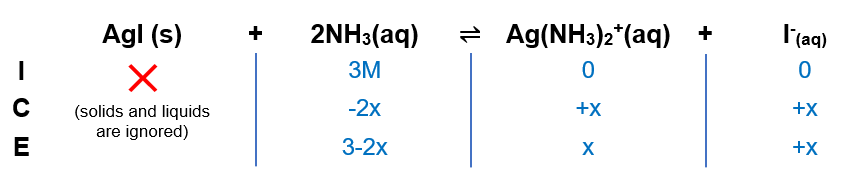# Problem: Calculate the molar solubility of AgI in 3.0 M NH 3. The overall formation constant for Ag(NH3)2+ is 1.7 X 107.

###### FREE Expert Solution

(1) Determine the Keq equation

AgI ⇌ Ag+ (aq) + I- (aq)                           Kf = 1.7x107

Ag+ + 2NH⇌ Ag(NH3)2+                               Ksp  = 1.5x1016

--------------------------------------

AgI (s) + 2 NH3 (aq) ⇋ Ag(NH3)2+  + I- (aq)        Keq = 1.5x1016 x 1.7x107 = 2.55x10-9

(2) Construct an ICE chart83% (44 ratings)###### Problem Details

Calculate the molar solubility of AgI in 3.0 M NH 3. The overall formation constant for Ag(NH3)2+ is 1.7 X 107.